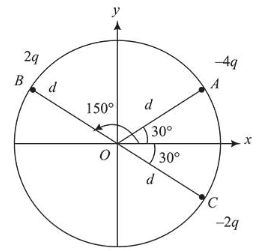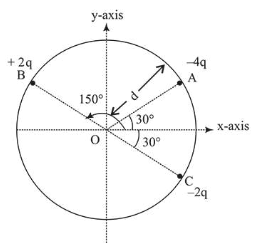# Three charged particles A, B and C with chargesQuestion:

Three charged particles $A, B$ and $C$ with charges $-4 q, 2 q$ and $-2 q$ are present on the circumference of a circle of radius $d$. The charged particles $A, C$ and centre $O$ of the circle formed an equilateral triangle as shown in figure. Electric field at $O$ along $x$-direction is:1. (1) $\frac{\sqrt{3 q}}{\pi \in_{0} d^{2}}$

2. (2) $\frac{2 \sqrt{3 q}}{\pi \in_{0} d^{2}}$

3. (3) $\frac{\sqrt{3 q}}{4 \pi \in_{0} d^{2}}$

4. (4) $\frac{3 \sqrt{3 q}}{4 \pi \in_{0} d^{2}}$

Correct Option: 1

Solution:

(1)Electric field due to charge $+2 q$ at centre $O$

$\vec{E}_{1}=\frac{1}{4 \pi \varepsilon_{0}} \times \frac{2 q}{d^{2}}\left[\frac{\sqrt{3} \hat{i}-\hat{j}}{2}\right]$

Electric field due to charge $-2 q$ at centre $O$

$\vec{E}_{2}=\frac{1}{4 \pi \varepsilon_{0}} \times \frac{2 q}{d^{2}}\left[\frac{\sqrt{3} \hat{i}-\hat{j}}{2}\right]$

Electric field due to charge $-4 q$ at centre $O$

$\vec{E}_{3}=\frac{1}{4 \pi \varepsilon_{0}} \times \frac{4 q}{d^{2}}\left[\frac{\sqrt{3} \hat{i}+\hat{j}}{2}\right]$

$\therefore$ Net electric field at point $O$

$\vec{E}_{0}=\vec{E}_{1}+\vec{E}_{2}+\vec{E}_{3}=\frac{\sqrt{3} q}{\pi \varepsilon_{0} d^{2}} \hat{i}$